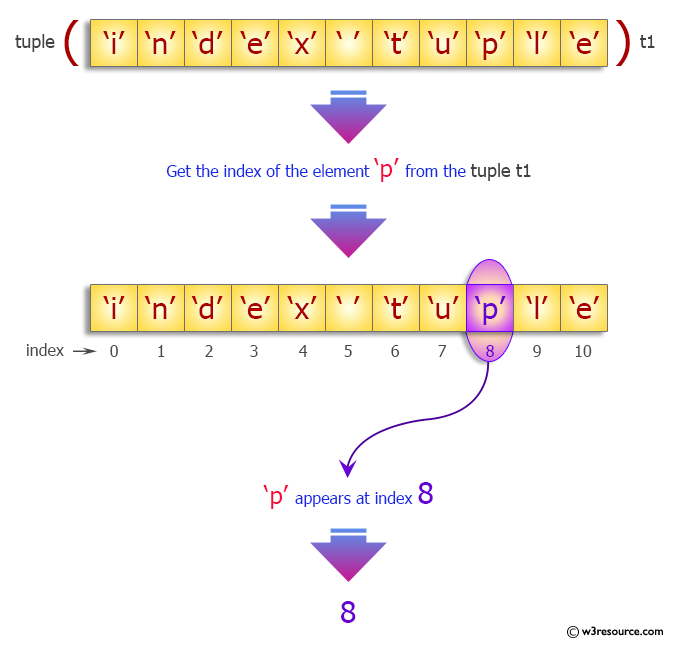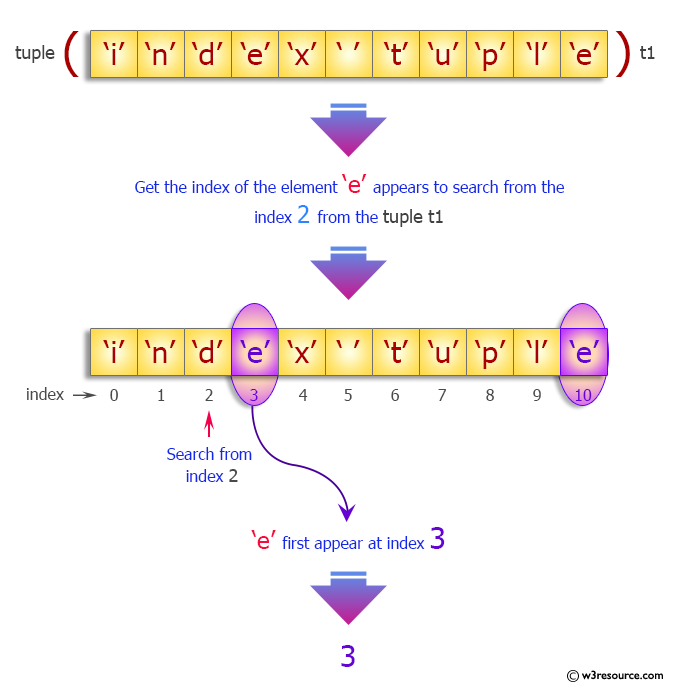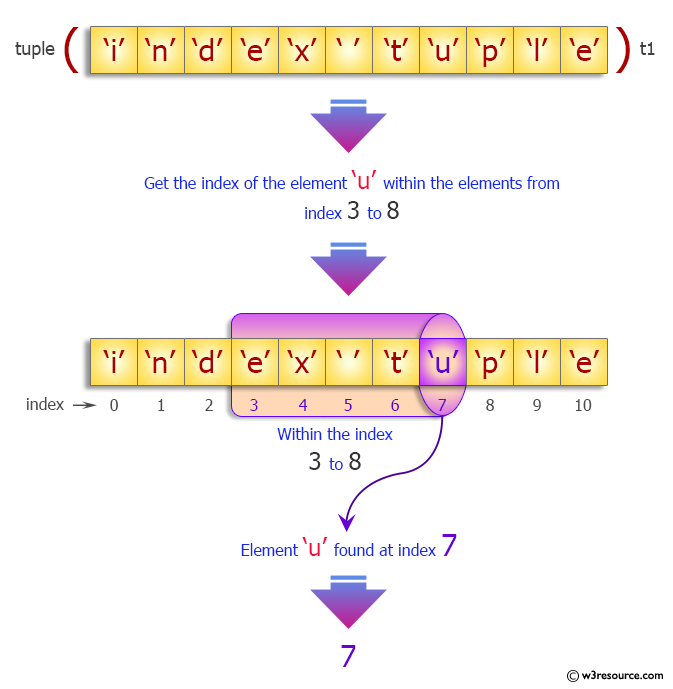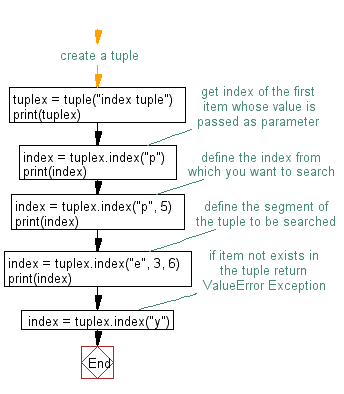﻿ Python: Find the index of an item of a tuple - w3resource# Python Exercise: Find the index of an item of a tuple

## Python tuple: Exercise-14 with Solution

Write a Python program to find the index of an item of a tuple.

Sample Solution:-

Python Code:

``````#create a tuple
tuplex = tuple("index tuple")
print(tuplex)
#get index of the first item whose value is passed as parameter
index = tuplex.index("p")
print(index)
#define the index from which you want to search
index = tuplex.index("p", 5)
print(index)
#define the segment of the tuple to be searched
index = tuplex.index("e", 3, 6)
print(index)
#if item not exists in the tuple return ValueError Exception
index = tuplex.index("y")
```
```

Sample Output:

```('i', 'n', 'd', 'e', 'x', ' ', 't', 'u', 'p', 'l', 'e')
8
8
3
Traceback (most recent call last):
File "d0e5ee40-30ab-11e7-a6a0-0b37d4d0b2c6.py", line 14, in <module>
index = tuplex.index("y")
ValueError: tuple.index(x): x not in tuple
```

Pictorial Presentation:Flowchart:## Visualize Python code execution:

The following tool visualize what the computer is doing step-by-step as it executes the said program:

Python Code Editor:

Have another way to solve this solution? Contribute your code (and comments) through Disqus.

What is the difficulty level of this exercise?

Test your Programming skills with w3resource's quiz.

﻿

## Python: Tips of the Day

Decapitalizes the first letter of a string:

Example:

```def tips_decapitalize(s, upper_rest=False):
return s[:1].lower() + (s[1:].upper() if upper_rest else s[1:])
print(tips_decapitalize('PythonTips'))
print(tips_decapitalize('PythonTips', True))
```

Output:

```pythonTips
pYTHONTIPS
```